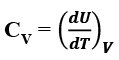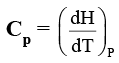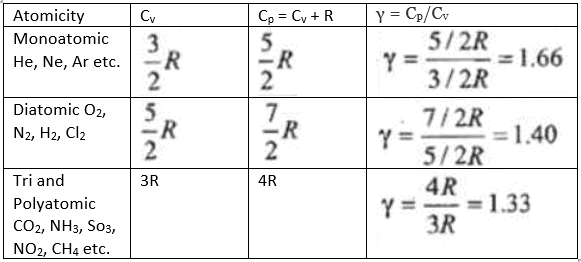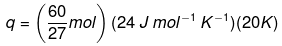Courses

# Types of Enthalpy, Heat Capacity and Specific Heat Capacity Class 11 Notes | EduRev

## Class 11 : Types of Enthalpy, Heat Capacity and Specific Heat Capacity Class 11 Notes | EduRev

The document Types of Enthalpy, Heat Capacity and Specific Heat Capacity Class 11 Notes | EduRev is a part of the Class 11 Course Chemistry Class 11.
All you need of Class 11 at this link: Class 11

ENTHALPY (H)
The quantity U + PV is known as the enthalpy of the system and is denoted by H. It represents the total energy stored in the system. Thus
H = U + PV

The absolute value of enthalpy can not be determined, however the change in enthalpy can be experimentally determined.
ΔH = ΔU + Δ(PV)

It is the sum of internal energy and pV-energy of the system. It is a state function and extensive property. Mathematically,
H = U + pV

Like U, absolute value of H also cannot be known, ΔH is determined experimentally.
ΔH = H2 – H1
or ΣHp = ΣHR

For exothermic reaction (the reaction in which heat is evolved), ΔH = -ve whereas for endothermic reaction (the reaction in which heat is absorbed), ΔH = +ve.

DERIVATION OF FORMULA
We may write equation ∆U=q + w as ∆U= qp -P∆V at constant pressure, where qp is heat absorbed by the system and –p∆V represent expansion work done by the system.

Let us represent the initial state by subscript 1 and final state by 2 We can rewrite the above equation as
U2 – U1 = qp – p (V2 – V1)

On rearranging, we get
qp = (U+ pV2) – (U1 + pV1) -----------(1)
Now we can define another thermodynamic function, the enthalpy H [Greek word enthalpien, to warm or heat content] as :
H = U + pV
so, equation (1) becomes
q= H2 – H1 = ΔH
ΔH = ΔU + pΔV     ...................(2)
Although q is a path dependent function, H is a state function because it depends on U, p and V, all of which are state functions.
Therefore, ∆H is independent of path i.e a State function . Hence, qp is also independent of path.

Relationship between ΔH and ΔU
ΔH = ΔU + Δp Δ V

Let us consider a reaction involving gases. If VA is the total volume of the gaseous reactants, VB is the total volume of the gaseous products, nA is the number of moles of gaseous reactants and nB is the number of moles of gaseous products, all at constant pressure and temperature, then using the ideal gas law, we write,
pVA = nART
and pVB = nBRT
Thus, pVB – pVA = nBRT – nART = (n– nA)RT
or p (VB – VA) = (nB – nA) RT
or p ∆V = ∆ngRT -----------(3)
Here, ∆ng refers to the number of moles of gaseous products minus the number of moles of gaseous reactants.
Substituting the value of p ∆V from equation (3) in equation (2), we get
= ∆+ ∆ngRT
It is important to note that when heat is absorbed by the system at constant pressure, we are actually measuring changes in the enthalpy.
Remember ΔH = qv, heat absorbed by the system at constant pressure.

SIGN CONVENTION OF ENTHALPY

• ΔH is negative for exothermic reactions which evolve heat during the reaction
• ΔH is positive for endothermic reactions which absorb heat from the surroundings.

At constant volume (ΔV = 0), ΔU = qV, therefore equation  becomes ΔH = ΔU = qV

HEAT CAPACITY OF A SYSTEM
Heat Capacity (c) of a system is defined as the amount of heat required to raise the temperature of a system by 1°C.
Let a very small quantity of heat dq be given to a system and the temperature of the system rises by dT.
Heat capacity = dq/dT

• The SI unit of heat capacity is joule per kelvin (J/K).
• Heat capacity is an extensive property.

MOLAR HEAT CAPACITY
Molar heat capacity is the amount of heat needed to raise the temperature of 1 mole of a substance by 1 Kelvin.
The SI unit of molar heat capacity is  J/mol/K

SPECIFIC HEAT CAPACITY
it is the amount of energy that must be added, in the form of heat, to one unit of mass of the substance in order to cause an increase of one unit in its temperature.

q = mc ΔT.
where, m = mass of substance

The heat capacity of a system, particularly in a gaseous system, determined at constant volume, is different from that determined at constant pressure.

• At constant volume qV = ΔU=CV ΔT
Molar sp. heat at constant volume• At constant pressure qp = ΔH=CP ΔT

Molar sp. heat at constant pressureFor a mole of an ideal gas,
ΔH = ΔU + Δ(pV)
= ΔU + Δ(RT)
= ΔU + RΔT
ΔH = ΔU+ R
On putting the values of ΔH and ΔU,
we have
CP ΔT = CV ΔT + R ΔT
CP ΔT - CV ΔT = R ΔT
ΔT (CP - CV ) = R ΔT

 CP - CV = R

(Cp and CV are specific heats at constant pressure and constant volume respectively)
Cp – CV = R (R = Molar gas constant)
The molar heat capacity at constant volume, CV = (3 / 2) R
The molar heat capacity at constant pressure,
Cp = (3 / 2) R + R = (5 / 2)R
Ratio of heat capacity γ = Cp / Cdepends on atomicity of gas.
Poisson’s ratio, γ = Cp / CV = (5 / 3) = 1.66
γ = 1.66 for monoatomic gas
γ = 1.40 for diatomic gas
γ = 1.33 for triatomic gasSOLVED EXAMPLES
Example 1. Calculate the energy needed to raise the temperature of 10 g of iron from 25 degree Celsius to 500 degree Celsius if specific heat capacity of iron is 0.45 J g -1˚C -1.

Solution: Here change in temp.=500 - 25=475 ˚C
Total heat = mass × Specific Heat Capacity × change in temp
= m × Cs × ∆T
=10 × 0.45 × 475
=2137.5 Joules

Example 2. Calculate the number of kJ of heat necessary to raise the temperature of 60.0 g of aluminium from 35°C to 55°C. Molar heat capacity of Al is 24 J mol–1 K–1.
Solution. From the expression of heat (q),
q = m. c. ΔT
Where,
c = molar heat capacity
m = mass of substance
ΔT = change in temperature
Substituting the values in the expression of q:q = 1066.7 J
q = 1.07 kJ

Offer running on EduRev: Apply code STAYHOME200 to get INR 200 off on our premium plan EduRev Infinity!

## Chemistry Class 11

136 videos|245 docs|180 tests

,

,

,

,

,

,

,

,

,

,

,

,

,

,

,

,

,

,

,

,

,

,

,

,

;